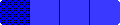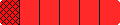Home
 .............Teaching Fraction Concepts Grades 5 to 8 .The eight files below are intended to provide teachers with ideas for teaching fractions in a way that will make sense to students. Usually instruction is centered around teaching rules for using fractions, but the purpose of the illustrations in the following files is to suggest ways to provide an intuitive understanding of fraction notation, equality, inequality, and the four basic operations. These illustrations will help show teachers how to make connections between the models and the algorithms for learning about fractions. These files also contain comments and suggestions for teachers as well as tables from NCTM's Standards and NAEP's research results which document some of the common difficulties students experience in learning about fractions. Students may find such tables interesting. There are also several samples of fifth grade student papers about fractions and their operations. Further samples and suggestions for writing in math are in the file Writing About Fractions. Introduction to Fraction Bars 5-Bars and Fraction Bingo (equality) Small Step Race (equality) Super FRIO (inequality) Capture (subtraction) Equations Game Fractions and Operations Linear Model - Number Lines The file Overhead Masters for Workshop can be used to make transparencies of the selected games and activities in these eight files. Transparent Fraction Bars are recommended for illustrating the games and activities. These can be ordered or produced from the abbreviated set of Fraction Bars masters.

HomeHome